# Simplifying Fractions

Reduce those clunky fractions down to something more manageable.Author
Taylor HartleyExpert Reviewer

Published: August 24, 2023# Simplifying Fractions

Reduce those clunky fractions down to something more manageable.Author
Taylor HartleyExpert Reviewer

Published: August 24, 2023# Simplifying Fractions

Reduce those clunky fractions down to something more manageable.Author
Taylor HartleyExpert Reviewer

Published: August 24, 2023Key takeaways

• Simplifying fractions makes them easier to work with – Reducing fractions makes them easier to visualize and work into other math problems.
• It’s all about the GCF – The greatest common factor (GCF) of both the numerator and denominator will help you figure out if the fraction is in its simplest form.
• 1 factor to rule them all – You’ll know that you have a simplified fraction if the GCF of both the numerator and the denominator is 1.

In your adventures with math, you might find yourself with a fraction like 2967/5934. Not only is it a bit of a mouthful, but it’s also hard to work with in additional math problems. This is where simplifying fractions comes in handy. Through the simplification process, something as big and clunky as 2967/5934 can be reduced down to 1/2! Now that is a much easier number to play with.

An important thing to keep in mind is that simplifying fractions does not change their value. It may seem like turning a bunch of big numbers like 2967/5934 into the seemingly smaller 1/2 would mean the fraction’s value is smaller, but that’s not the case at all. In both fractions, the top number (the numerator) is exactly half of the bottom number (the denominator). Both fractions represent the value of one half. All we’ve done is make the way the value is represented more manageable.

It may also help to visualize this a bit. Think about slicing up a birthday cake. You can slice the cake into 100 pieces or 4 pieces, but you still have the same amount of cake.  That is why 25 pieces of the 100 piece cake is the exact same as 1 piece of the 4 piece cake. One big piece of cake is easier to eat than 25 tiny pieces, right? That’s why simplifying fractions is so helpful.

## How to simplify fractionsBy the end of the simplification process, the goal is that the greatest common factor (between the numerator and the denominator) is 1. Remember, a factor is a perfectly divisible number. This means you can divide a factor by another number and have no remainders. For example, the factors of 12 are 1, 2, 3, 4, 6, and 12. This is because you can perfectly divide 12 by all those numbers.

Therefore, in order to simplify a fraction, you will need to find the factors of both the numerator and the denominator.

### Find the factorsLet’s work with the fraction 12/36.

To begin simplifying, we need to find the factors of both the numerator (12) and the denominator (36). The simplest way to do this is to start with 1 and begin dividing the original number by larger and larger numbers until the quotient (the answer to the division problem) is 1. Any quotients you get that are whole numbers mean that the divisor (the number you are currently dividing by) is a factor.

For 12, we can start by dividing by 1, which gets us a quotient of 12. All numbers are divisible by 1, so that’s a freebie. We then move on to 2, which gets us 6. This is a whole number, so 2 is a factor. And so on.

When you finish this process for both 12 and 36, you will find the following factors:

12 has factors of 1, 2, 3, 4, 6, and 12

36 has factors of 1, 2, 3, 4, 6, 9, 12, 18, and 36

When you look at these two lists of factors, you can see that, for both 12 and 36, the greatest common factor (GCF) is 12. Keep this GCF handy because you’ll need it for the next step!

Finding factors can take a little bit of time. Luckily, there are a few tricks to speed up some parts of the process..

• All numbers are divisible by 1 and itself
• If the number is even, then it is divisible by 2
• If you add up each digit and that number is divisible by 3 (for example 18: 1 + 8 = 9), then the number is divisible by 3.
• If the ones digit is a 0 or a 5, then it is divisible by 5.

### Divide by the GCFNow that you have the GCF, which is 12 in this case, all you need to do is divide the numerator and the denominator by that number. Remember, we are trying to simplify the fraction 12/36.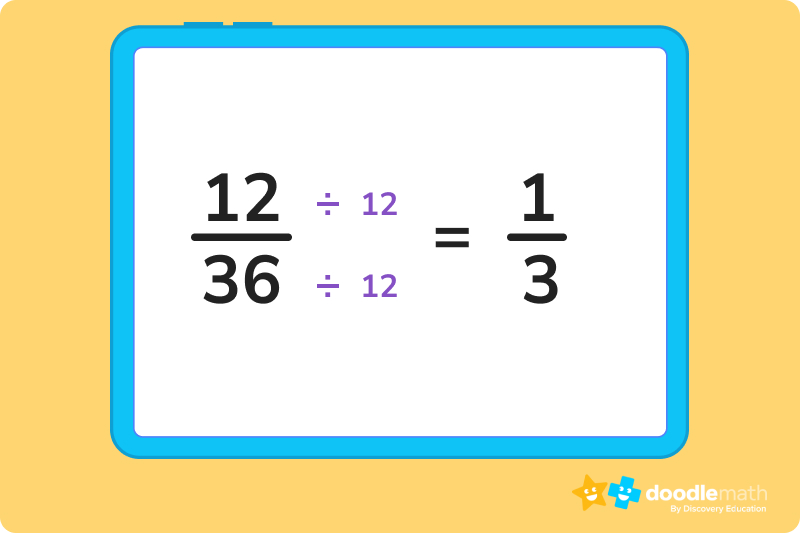This leaves us with the simplified fraction of 1/3!

Check your work by factoring out the numerator and denominator again! This makes sure that they do not have any common factors larger than 1.

## How to convert improper fractions to mixed numbersWhen the numerator is larger than the denominator, you have an improper fraction on your hands. Let’s learn how to clean that up!

For example, we might have the fraction 23/6. 23 is obviously larger than 6, so we need to reduce it.

The first step is to divide the numerator by the denominator. This is 23 ÷ 6, which gets us 3 with a remainder of 5. If you need help getting this answer, make sure to check out our how-to guide on division.

Next, you take the whole number quotient (3) and put it in front of the fraction. You then take the remainder (5) and put that over the original denominator (6). This gives you the mixed fraction of 3 5/6.

## How to simplify mixed fractionsFortunately, simplifying mixed fractions is nice and simple. You can basically ignore the whole number on the left and simplify the fraction as explained before. Let’s take a look at an example.

We have the mixed fraction 2 15/45, which clearly needs to be simplified. Now, how do we do this? Let’s start by putting that 2 to the side and factoring out the numerator and denominator of the remaining fraction.

15 has factors of 1, 3, 5, and 15

45 has factors of 1, 3, 5, 9, 15, and 45

The GCF between those two sets is 15, so we will divide both the numerator and the denominator by 15.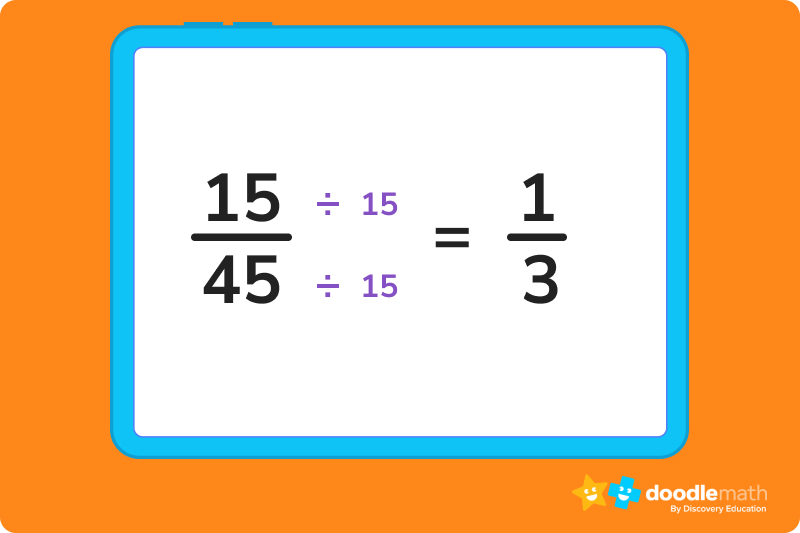Now we bring the whole number back in, and, voila, we have our simplified mixed fraction: 2 1/3!

## Let’s practice together!### 1. Simplify the fraction 12/24When we factor out both the 12 and the 24, the GCF is 12. When we divide both by 12, we get the simplified fraction of 1/2.

### 2. Convert the fraction 15/4 to a mixed number.Since the numerator is greater than the denominator, this is an improper fraction. We need to first divide the numerator by the denominator, which will give us 3 with a remainder of 3. We then pop that 3 over the denominator 4 to end up with the mixed fraction of 3 3/4.

### 3. Convert the mixed fraction 3 1/2 into an improper fraction and then simplify.This might seem a little tricky, but think, you just have to work in reverse. Instead of dividing numbers, multiply them! Multiply the denominator by the whole number and then add that to the numerator. That will get you 7/2. Now, check to see if there is a GCF greater than 1. Looks like the GCF of both 7 and 2 is 1. This means 7/2 is the simplest version of this improper fraction.

Ready to give it a go?

You now have the basics needed to tackle some of the more common fraction simplification problems. Dig into the following questions and test your understanding. Remember that you can also look back at the guide if you bump into any problems.

## Parent Guide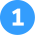How did we get here?

You can go through the process of factoring. 10 ⮞ 1, 2, 5, and 10. 50 ⮞ 1, 2, 5, 10, 25, and 50. The GCF is 10, and when you divide both the numerator and denominator, you get 1/5.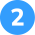How did we get here?

Go through the process of factoring. 21 ⮞ 1, 3, 7, and 21. 63 ⮞ 1, 3, 7, 9, 21, and 63. The GCF is 21, and when you divide both the numerator and denominator, you get 1/3.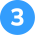How did we get here?

1. Start by dividing the numerator by the denominator. 27 ÷ 8 = 3 with a remainder of 3.
2. Now take the remainder and place it as the new numerator over the original denominator. This gets you 3/8.
3. Lastly, put that fraction next to the 3 you got by dividing the numerator by the denominator. You now have 3 3/8.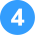How did we get here?

1. Start by dividing the numerator by the denominator. 33 ÷ 11 = 3.
2. This gives you a whole number, so you are all done! The answer is 3.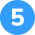How did we get here?

1. Start by multiplying the whole number by the denominator. 5 X 4 = 20.
2. Now add that 20 to the existing numerator. 20 + 3 = 23.
3. You now have 23/4! Double check that this is the simplest form by factoring out and looking for a GCF greater than 1. Since there isn’t one, this fraction is as simple as possible while still being an improper fraction.

## FAQs about math strategies for kidsWe understand that diving into new information can sometimes be overwhelming, and questions often arise. That’s why we’ve meticulously crafted these FAQs, based on real questions from students and parents. We’ve got you covered!

Look for the greatest common factor (GCF) of the numerator and denominator and then divide both by that number.

Ignore the whole number and just focus on the fraction. Go through the same process of simplification that you’d use on a regular fraction.

Use some tricks to reduce the numbers into more manageable options. If both numbers are even, you can go ahead and divide by 2. If the digits in each number add up to a multiple of 3, you can divide both by 3. If both numbers end in 0 or 5, you can divide them both by 5. From there, you can easily factor out the smaller numbers.

You will need to reduce the improper fraction into a mixed fraction. Divide the numerator by the denominator and set that number aside. Use any remainder as the new numerator for your fraction. Pop that above the existing denominator and then simplify the fraction, if necessary. Now you have a simplified mixed fraction.## Related Posts

Title 1

Title 2

Title 3

Lesson credits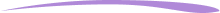Taylor Hartley

Taylor Hartley is an author and an English teacher based in Charlotte, North Carolina. When she's not writing, you can find her on the rowing machine or lost in a good novel.

Jill Padfield has 7 years of experience teaching high school mathematics, ranging from Alegra 1 to AP Calculas. She is currently working as a Business Analyst, working to improve services for Veterans while earning a masters degree in business administration.

Taylor Hartley

Taylor Hartley is an author and an English teacher based in Charlotte, North Carolina. When she's not writing, you can find her on the rowing machine or lost in a good novel.

Jill Padfield has 7 years of experience teaching high school mathematics, ranging from Alegra 1 to AP Calculas. She is currently working as a Business Analyst, working to improve services for Veterans while earning a masters degree in business administration.# Are you a parent, teacher or student?

Are you a parent or teacher?

## Hi there!

Book a chat with our team